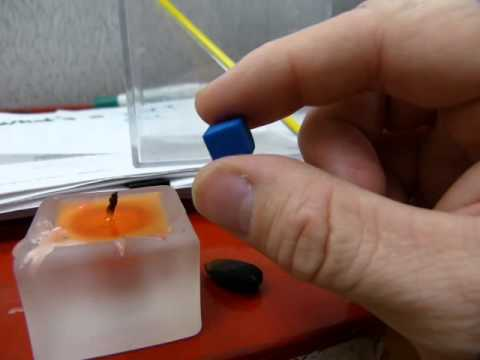### Main Difference

Cal and Kcal are the units of energy. cal stands for calories while kcal stands for kilocalories. cal is the smaller unit of energy while kcal is the large unit of energy. 1 kcal is equal to 1000 cal. cal is the amount of energy or heat needed to raise the temperature of 1 g of water by 1 degree Celsius while kcal is the amount of energy or heat needed to raise the temperature of 1 kg of water by 1 degree Celsius.

### What is Cal?

Cal is the unit of energy stands for calories. It is the amount of energy or heat needed to raise the temperature of 1 g of water by 1 degree Celsius. cal is mostly used for the energy stored in the food items. One calorie is equal to 4.184 Joules. 1gram of protein carries 4 cal of energy and one gram of fat carries 9 cal of energy.

### What is Kcal?

Kcal is the unit of energy stands for kilocalories. It is the amount of energy or heat needed to raise the temperature of 1 kg of water by 1 degree Celsius. kcal is mostly used for the energy stored in the food item. One kcal is equal to 4184 Joules.

### Key Differences

1. cal is smaller unit of energy while kcal is the larger unit of energy.
2. cal is the amount of energy or heat needed to raise the temperature of 1 g of water by 1 degree Celsius while kcal is the amount of energy or heat needed to raise the temperature of 1 kg of water by 1 degree Celsius.
3. One cal is equal to 4.184 Joule while one kcal is equal to 4184 Joules.
4. cal stands for calories and kcal stands for kilocalories.
5. 1 gram of carbohydrates contains 4 calories of energy while 1 gram of carbohydrates contains 0.004 kilocalories of energy.
6. 1 gram of fat contains 9 calories of energy while 1 gram of fat contains 0.009 kilocalories of energy.
7. 4 liters of gasoline contains 31000000 calories while 4 liters of gasoline contains 31000 kilocalories.
8. 1 gram of protein contains 4 calories of energy while 1 gram of protein contains 0.004 kilocalories of energy.